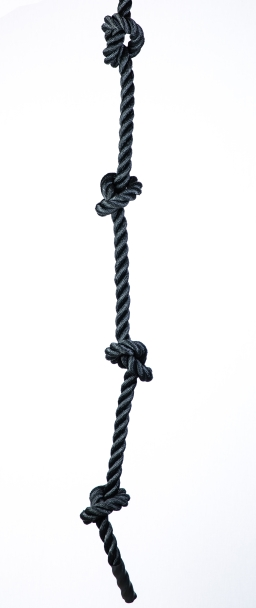# Carl bought

Carl bought 36 m. Of rope. He wants to divide it equally into 1 3/5 m. Each. How many shorter ropes can he make?

n =  22

### Step-by-step explanation:Did you find an error or inaccuracy? Feel free to write us. Thank you!

Tips for related online calculators
Need help calculating sum, simplifying, or multiplying fractions? Try our fraction calculator.
Need help with mixed numbers? Try our mixed-number calculator.
Do you want to convert length units?